﻿ 轨道车辆轮对模态试验仿真验证分析 Modal Testing and Simulation Verification of Rail Vehicle Wheelset

Open Journal of Transportation Technologies
Vol. 09  No. 03 ( 2020 ), Article ID: 35689 , 9 pages
10.12677/OJTT.2020.93018

Modal Testing and Simulation Verification of Rail Vehicle Wheelset

Changlong Zhao, Xiaoliang Dou

CRRC Qingdao Sifang Co., Ltd., Qingdao Shandong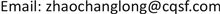Received: May 1st, 2020; accepted: May 14th, 2020; published: May 21st, 2020ABSTRACT

Based on PolyMAX modal identification algorithm and hammer excitation method, the modal parameters of the wheelset of rail vehicles under different boundary conditions are tested, and the influence of track constraints on the typical modal frequencies of the wheelset is compared and analyzed. On this basis, the finite element simulation model of the wheelset is established; the modal frequencies and modal shapes of the wheelset under the free boundary is calculated and verified with the test results. The results show that the simulation results of the modal test of the wheelset are in good agreement. The modeling method and finite element model of the wheelset can be used for the rapid prediction of the vibration modal matching in the vehicle design and optimization stage.

Keywords:Wheelset, Modal Testing, Modal Simulation, Boundary Condition, Finite Element Simulation1. 引言

2. 轮对模态试验

2.1. 试验方法

2.2. 试验数据校验

(1) 频响校验：

(2) 相干性校验：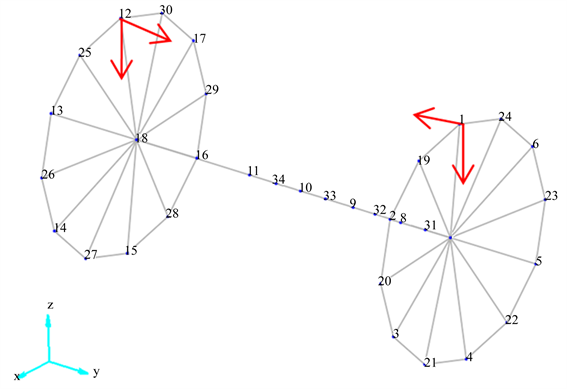Figure 1. Geometry model of the wheelset for modal test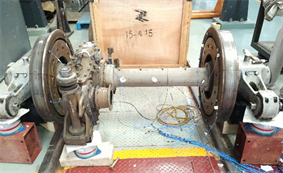(a)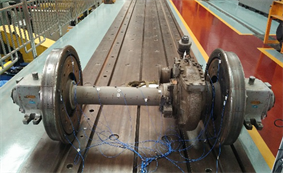(b)

Figure 2. Field layout of wheelset modal test. (a) Elastic support; (b) Rail-constraint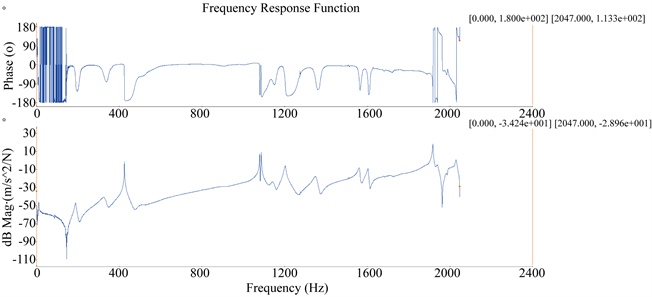Figure 3. Typical frequency response function (phase and amplitude)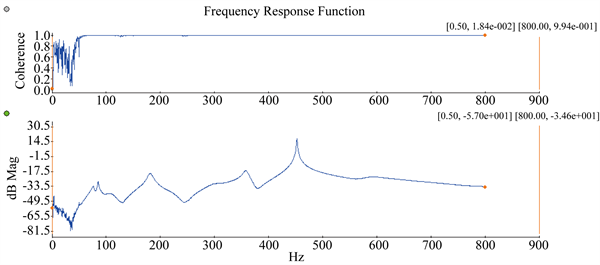Figure 4. Coherence function and amplitude of FRF

(3) 互异性校验：Figure 5. Coincident validation of FRF

2.3. 模态辨识结果Table 2. Rigid body modal frequencies and modal shapes of the wheelset under track-constraint

3. 模态参数的数值求解与相关性分析方法

3.1. 模态参数的数值求解方法

$\left[M\right]\left\{\stackrel{¨}{u}\right\}+\left[K\right]\left\{u\right\}=\left\{0\right\}$ (1)

$\left\{u\right\}={\left\{A\right\}}_{n}\mathrm{sin}\left(\omega t+\phi \right)$ (2)

$\left(\left[K\right]-{\omega }_{n}^{2}\left[M\right]\right){\left\{A\right\}}_{n}=\left\{0\right\}$ (3)

$\left\{A\right\}$ 有非零解的充要条件是系数行列式等于零，即：

$|\left[K\right]-{\omega }^{2}\left[M\right]|=|0|$ (4)

$\left(\left[K\right]-{\omega }_{n}^{2}\left[M\right]\right){\left\{\text{Φ}\right\}}_{n}=\left\{0\right\}$ (5)

3.2. 模态参数的相关性分析

$MA{C}_{ij}=\frac{{|\left({\text{Φ}}_{i}^{eT}{\text{Φ}}_{j}^{a}\right)|}^{2}}{\left({\text{Φ}}_{i}^{eT}{\text{Φ}}_{i}^{e}\right)\left({\text{Φ}}_{j}^{aT}{\text{Φ}}_{j}^{a}\right)}$ (6)

4. 轮对自由模态试验仿真分析

4.1. 轮对有限元数值模型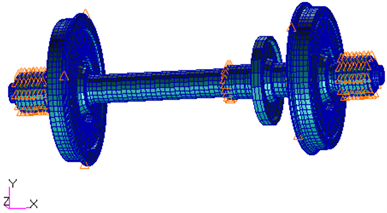Figure 6. Finite element model of wheelset

4.2. 试验仿真校验Table 4. Matching results of test and numerical modal

Table 5. Modal identification results of wheelset

5. 结语

Modal Testing and Simulation Verification of Rail Vehicle Wheelset[J]. 交通技术, 2020, 09(03): 150-158. https://doi.org/10.12677/OJTT.2020.93018

1. 1. 窦晓亮. 轨道车辆地板组合断面模态试验仿真验证分析[J]. 商情, 2019(14): 201-202.

2. 2. 曹研研, 赵登峰. 有限元模态分析理论及其应用[J]. 制工程与自动化, 2007(2): 73-74.

3. 3. 吴东亚, 刑宏光, 崔军, 等. 基于有限元的某型坦克炮身管模态分析[J]. 科技导报, 2008, 26(23): 44-47.

4. 4. 王彤, 张令弥. 运行模态分析的频域空间域分解法及其应用[J]. 航空学报, 2006, 27(1): 62-63.

5. 5. 孙鑫晖, 郝木明, 王潍潍. PolyMAX模态参数识别算法的快速实现[J]. 振动与冲击, 2011, 30(10): 6-8.

6. 6. 宋纪侠, 王彦, 章睿, 等. 白车身模态试验与模态仿真对标研究[J]. 汽车科技, 2016(4): 83-86.

7. 7. 包键, 宋俊, 毕锦烟, 等. 试验仿真模态误差分析[J]. 汽车实用技术, 2015(6): 56-58, 62.

8. 8. 刘盼, 夏汤忠, 王萍萍, 等. 轿车车身模态及扭转刚度灵敏度分析[J]. 汽车科技, 2011(6): 42-45.

9. 9. 徐茂林, 胡溧. 某型薄板冲压件骨架式白车身结构及模态分析[J]. 汽车科技, 2013(2): 53-56.